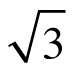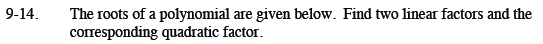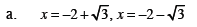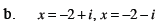Home > CCA2 > Chapter 9 > Lesson 9.1.1 > Problem9-14

9-14.
1. The roots of a polynomial are given below. Find two linear factors and the corresponding quadratic factor. Homework Help ✎

1. x = −2 +, x = −2 −2. x = −2 + i, x = −2 − iFind the first factor.

$(x - (-2 + \sqrt{3}))$

Find the second factor.

Multiply together to get the quadratic factor.Follow similar steps in part (a).